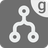Merge Intervals (generator version)
Undefined
English

You are given a sequence of intervals, as tuples of ints where the tuples are sorted by their first element in ascending order.
Your task is to minimize the number of intervals by merging those that intersect or by removing those that are a part of other intervals.
In this mission you should use 'yield' to make your function a generator.

Since the range of values for the intervals is restricted to integers, you must also merge those intervals between which no value can be found.

An example:
Let's say you've got these 5 intervals: 1..6, 3..5, 7..10, 9..12 and 14..16.

1. 1..6 and 3..5
3..5 fits into 1..6, so 3..5 must disappear.
2. 1..6 and 7..10
Even though the intervals don’t intersect, there isn’t an int type value between them, so they have to be merged with the new interval 1..10.
3. 1..10 and 9..12
These intervals do intersect, because 9 < 10, so they have to be merged with the new interval 1..12.
4. 1..12 and 14..16
These two intervals don’t intersect, so they mustn’t be merged.
So the intervals to be returned are:
1..12 and 14..16

Input: A sequence of intervals as a list of tuples of 2 ints, sorted by their first element.

Output: The merged intervals as a list of tuples of 2 ints, sorted by their first element.

Examples:

```merge_intervals([(1, 4), (2, 6), (8, 10), (12, 19)]) == [(1, 6), (8, 10), (12, 19)]
merge_intervals([(1, 12), (2, 3), (4, 7)]) == [(1, 12)]
merge_intervals([(1, 5), (6, 10), (10, 15), (17, 20)]) == [(1, 15), (17, 20)]```

Precondition:

• intervals == sorted(intervals, key=lambda x: x) # sorted by 1st element of the tuples
• interval <= interval